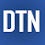## Pages

### Regression Model Accuracy (MAE, MSE, RMSE, R-squared) Check in R

Evaluating the model accuracy is an essential part of the process in creating machine learning models to describe how well the model is performing in its predictions. Evaluation metrics change according to the problem type. In this post, we'll briefly learn how to check the accuracy of the regression model in R.

The linear model (regression) can be a typical example of this type of problem, and the main characteristic of the regression problem is that the targets of a dataset contain the real numbers only. The errors represent how much the model is making mistakes in its prediction. The basic concept of accuracy evaluation is to compare the original target with the predicted one according to certain metrics.

Regression model evaluation metrics

The MSE, MAE, RMSE, and R-Squared metrics are mainly used to evaluate the prediction error rates and model performance in regression analysis.
• MAE (Mean absolute error) represents the difference between the original and predicted values extracted by averaged the absolute difference over the data set.
• MSE (Mean Squared Error) represents the difference between the original and predicted values extracted by squared the average difference over the data set.
• RMSE (Root Mean Squared Error) is the error rate by the square root of MSE.
• R-squared (Coefficient of determination) represents the coefficient of how well the values fit compared to the original values. The value from 0 to 1 interpreted as percentages. The higher the value is, the better the model is.
The above metrics can be expressed,
Calculating in R

We can calculate the above metrics manually and using the predefined function in R. We'll create two vector data to use in this tutorial.  The 'original' vector is original data and the 'predicted' vector is predicted by the model.

```original = c( -2,  1, -3, 2, 3, 5, 4, 6, 5, 6, 7)
predicted = c(-1, -1, -2, 2, 3, 4, 4, 5, 5, 7, 7)```

We can visualize it in a plot to check the difference visually.

```x=1:length(original)
plot(x, original,pch=19, col="blue")```
```lines(x, predicted, col="red")
legend("topleft", legend = c("y-original", "y-predicted"),
col = c("blue", "red"), pch = c(19,NA), lty = c(NA,1),  cex = 0.7)```

Next, we'll calculate the MAE, MSE, RMSE, and R-squared by applying the above formula.

```d = original-predicted
mse = mean((d)^2)
mae = mean(abs(d))
rmse = sqrt(mse)
R2 = 1-(sum((d)^2)/sum((original-mean(original))^2))

cat(" MAE:", mae, "\n", "MSE:", mse, "\n", ```
`    "RMSE:", rmse, "\n", "R-squared:", R2)`
` `
``` MAE: 0.6363636
MSE: 0.8181818
RMSE: 0.904534
R-squared: 0.9173623```

We can use R native and the 'caret' package's predefined functions to calculate the metrics.

`library(caret)`
` `
```# R native funcitons
MAE(predicted, original)```
```MSE(predicted, original)
```
`  0.6363636`
```  0.8181818

# caret package functions
RMSE(predicted, original)
 0.904534```
` 0.9173623`

The results are the same in both methods.

In this post, we've learned how to calculate regression accuracy measurements MAE, MSE, RMSE, and R-squared in R. The source code is listed below.

Source code listing

``````
library(caret)

original = c( -2,  1, -3, 2, 3, 5, 4, 6, 5, 6, 7)
predicted = c(-1, -1, -2, 2, 3, 4, 4, 5, 5, 7, 7)
x = 1:length(original)

plot(x, original,pch=19, col="blue")
lines(x, predicted,col="red")
legend("topleft", legend = c("y-original", "y-predicted"),
col=c("blue", "red"), pch=c(19,NA), lty=c(NA,1), cex = 0.7)
d = original-predicted
mse = mean((d)^2)
mae = mean(abs(d))
rmse = sqrt(mse)
R2 = 1-(sum((d)^2)/sum((original-mean(original))^2))

cat(" MAE:", mae, "\n", "MSE:", mse, "\n",
"RMSE:", rmse, "\n", "R-squared:", R2)

# R native funcitons
fmse = MSE(predicted, original)
fmae = MAE(predicted, original)

# caret package functions
frmse = RMSE(predicted, original)
fr2 = R2(predicted, original, form = "traditional")

cat(" MAE:", fmae, "\n", "MSE:", fmse, "\n",
"RMSE:", frmse, "\n", "R-squared:", fr2)

``````

1.Hai, i want to ask, can you give me the preferences that you use in this post?
Thankyou

1.Preferences? Sorry, I did not understand what you mean.
If it is about references that I used in this post, I can tell you that there are so many information and resource for this topic that I can't mention all of them. You can use Wikipedia and any book related to machine learning as a reference.

2.oh yaa, sorry i mean references.

2.chi squared?

3.Clear yet simple explanation. Appreciate. :-)

4.I'd like to ask why I have the error of "could not find function "MSE"

1.It looks MSE is not available in standard libraries.
You can use mltools package instead.

> install.packages("mltools")
> mltools::mse(2.323,2.789)
 0.217156

5.Gracias por la información